### Convergence Measures

The NLIN procedure computes and reports four convergence measures, labeled R, PPC, RPC, and OBJECT.

R

is the primary convergence measure for the parameters. It measures the degree to which the residuals are orthogonal to the columns of, and it approaches 0 as the gradient of the objective function becomes small. R is defined as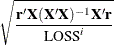PPC

is the prospective parameter change measure. PPC measures the maximum relative change in the parameters implied by the parameter-change vector computed for the next iteration. At the kth iteration, PPC is the maximum over the parameters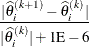where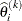is the current value of the ith parameter and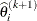is the prospective value of this parameter after adding the change vector computed for the next iteration. These changes are measured before step length adjustments are made. The parameter with the maximum prospective relative change is displayed with the value of PPC, unless the PPC is nearly 0.

RPC

is the retrospective parameter change measure. RPC measures the maximum relative change in the parameters from the previous iteration. At the kth iteration, RPC is the maximum over i of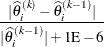whereis the current value of the ith parameter and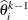is the previous value of this parameter. These changes are measured before step length adjustments are made. The name of the parameter with the maximum retrospective relative change is displayed with the value of RPC, unless the RPC is nearly 0.

OBJECT

measures the relative change in the objective function value between iterations: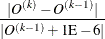where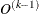is the value of the objective function (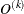) from the previous iteration. This is the old CONVERGEOBJ= criterion.